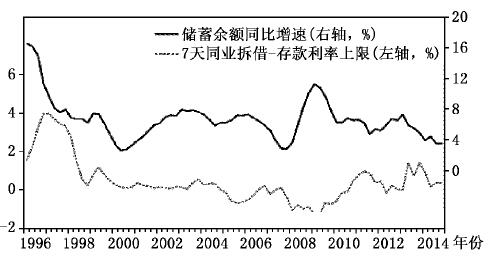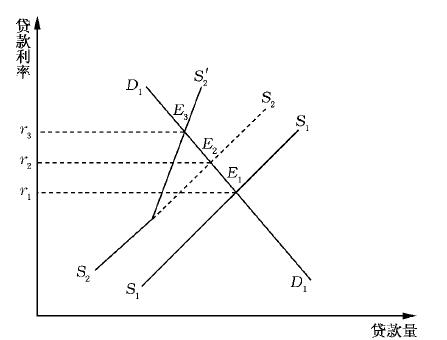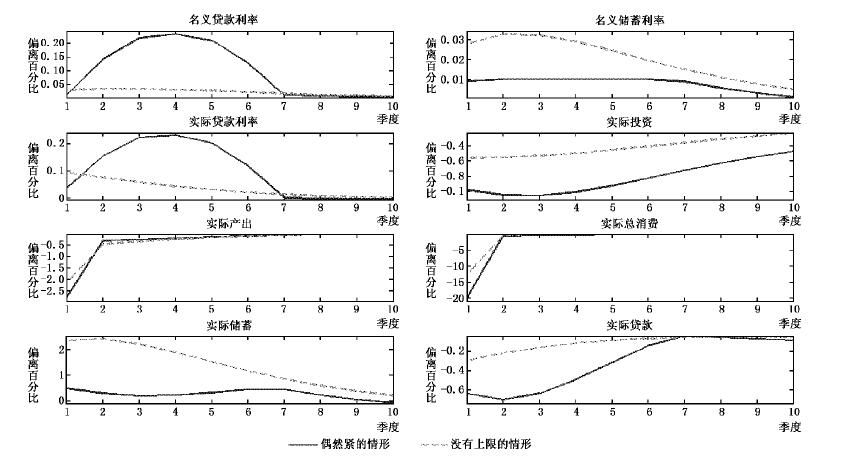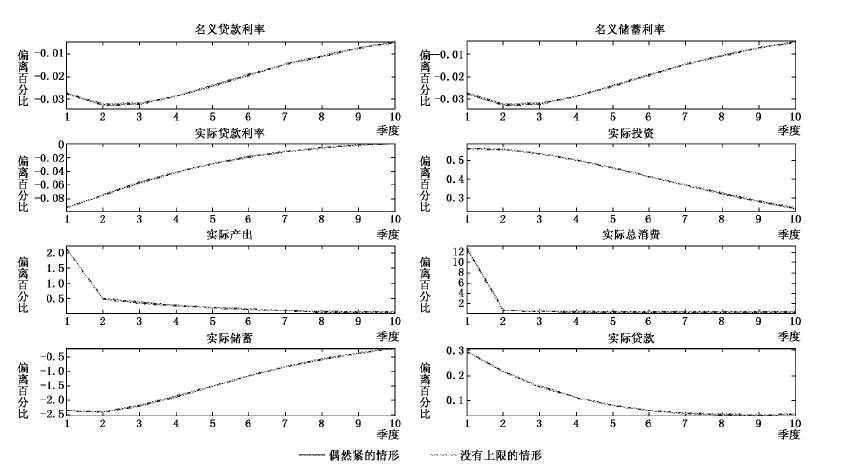﻿ 存款利率上限、金融脱媒与货币政策传导
 财经研究2016, Vol. 42Issue (1): 83-92,1330

#### 文章信息

Yang Fei

Deposit Rate Ceiling, Financial Disintermediation and Monetary Policy Transmission

Journal of Finance and Economics, 2016, 42(1): 83-92,133.

### 文章历史

Deposit Rate Ceiling, Financial Disintermediation and Monetary Policy Transmission
Yang Fei
School of Economics, Shanghai University of Finance and Economics, Shanghai 200433, China
Abstract: This paper introduces collateral loan constraints and deposit rate ceiling into a DSGE model and figures out how the deposit rate ceiling affects the monetary policy transmission.According to the actual circumstance,it allows deposit rate changes under the ceiling,and considers the occasionally binding case,which is ignored by most related literature.It arrives at the following conclusions:firstly,when the central bank tights the monetary policy,the deposit rate ceiling inhibits the rise in the deposit rates,leading to lower deposit rates,the reduction in household bank deposits and thereby financial disintermediation;secondly,financial disintermediation results in the decrease in banks'loanable funds,the distortion of loan supply,the increase in loan rates by a big margin and bigger economic fluctuations compared to the situation without deposit rate ceiling;thirdly,the occasionally binding ceiling exhibits some non-linearity that makes the impulse response of main economic variables to expansive and tight monetary policy shocks asymmetric.
Key words: deposit rate ceiling    monetary policy shock    occasionally binding constraint    loan supply distortion

①来自中国人民银行网站“新闻发布”和“货币政策”栏。本文完稿之后，中国人民银行于2015年5月11日将存款利率上浮区间调整至1.5倍，2015年8月26日放开1年期以上（不含1年期）定期存款的利率浮动上限，2015年10月24日起全面放开存款利率上限。图 1 银行间7天同业拆借利率和存款利率上限的利差与居民储蓄余额增速比较

② 本文考虑狭义的金融脱媒，即在存款利率上限管制下，当市场利率高于存款机构可支付的存款利率水平时，存款机构储蓄流失的现象。更多的定义可参见宋旺和钟正生（2010）。

①2012年6月8日之前的存款利率上限是中国人民银行公布的1年期定期存款基准利率，之后的是央行公布的基准利率上浮到顶的情形。居民储蓄余额数据来自Wind资讯，银行间7天同业拆借利率来自中经网统计数据库，基准利率及调整幅度来自中国人民银行网站和Wind资讯。

②本文的研究思路受Mertens(2008)启发，但模型构建、模型机制和求解方法均有很大不同，限于篇幅，具体比较可向作者索取。图 2 银行间7天同业拆借利率与1年期定期存款利率上限比较②

1996年1月至2015年2月银行间7天同业拆借加权平均利率来自中经网统计数据库，存款利率上限的处理同图1。

①这一结论对于文献中常用的市场利率都适用。我们利用时间跨度略短的上海银行间7天同业拆借利率和银行间债券回购7天利率，可以得到高度类似的结果。图 3 贷款供求分析

① 我国贷款利率在历史上也存在管制，但与存款利率管制相比，贷款利率管制很早就开始放松，且2013年全面放开对金融机构的贷款利率管制。

(一)家庭部门

 ${E_0}\sum\limits_{t = 0}^\infty {{\beta ^t}\left[ {\ln \left( {{c_t}} \right) + \varphi \ln \left( {{Q_t}/{P_t}} \right) - \xi n_t^{1 + \phi }/\left( {1 + \phi } \right)} \right]}$ (1)

② 是否有消费习惯并不影响本文的主要结论，加入消费习惯的情形可向作者索取。

 ${c_t} + {d_t} + {q_t} - {q_{t - 1}}/{\pi _t} \leqslant {w_t}{n_t} + R_{t - 1}^d{d_{t - 1}}/{\pi _t} + f_t^r + f_t^b$ (2)

(二)生产部门

1.企业家。无限期生存的代表性企业家拥有中间产品生产企业，购买资本、雇用劳动和向银行借贷进行中间产品生产，以最大化其期望效用：
 ${E_0}\sum\limits_{t = 0}^\infty {\beta _e^t\ln \left( {c_t^e} \right)}$ (3)

 $c_t^e + {R_{t - 1}}{b_{t - 1}}/{\pi _t} + {w_t}{n_t} + q_t^k{k_t} + \varphi \left( {{u_t}} \right){k_{t - 1}} = {y_t}/{x_t} + {b_t} + q_t^k\left( {1 - \delta } \right){k_{t - 1}}$ (4)

 ${R_t}{b_t} \leqslant \tau {E_t}\left[ {q_{t + 1}^k{\pi _{t + 1}}\left( {1 - \delta } \right){k_t}} \right]$ (5)

τ<1表示贷款资本比率，银行给企业家的贷款量不会超过企业家所拥有资本的预期价值折现值的一定比例。

2.资本生产企业。市场上存在完全竞争的资本生产企业，同样为企业家所有。资本生产企业以价格qtk 从企业家手中获得折旧后的资本(1－δ)kt－1，并购买it单位最终产品来生产资本kt，再以价格qtk 卖给企业家。资本净产出为Δzt=kt－(1－δ)kt－1，资本生产企业选择it来最大化${E_0}\sum\limits_{t = 0}^\infty {\beta _e^t\Lambda _{0,t}^e\left( {q_t^k\Delta {z_t} - {i_t}} \right)}$，其中Λ0,te=c0e /cte

① 本文引入资本生产企业是为了构造抵押贷款约束时可以用资本的市值来抵押，事实上如果将资本投资积累直接融合到中间产品生产企业中，最终的最优化条件是一样的。

3.零售商。单位区间上存在连续的零售商，零售商j∈[0,1]以价格Ptw 购买中间产品，无成本地将1单位中间产品转化成异质的1单位最终产品j，最终产品组合采用Dixit Stiglitz加成${y_t} = {\left[ {\int_0^1 {{y_t}{{\left( j \right)}^{\left( {\varepsilon - 1} \right)/\varepsilon }}dj} } \right]^{\varepsilon /\left( {\varepsilon - 1} \right)}}$，其中ε是最终产品之间的替代弹性。求解成本最小化问题，则产品j的需求为yt(j)=(Pt(j)/Pt)－εyt，其中${P_t} = {\left[ {\int_0^1 {{P_t}{{\left( j \right)}^{1 - \varepsilon }}dj} } \right]^{1/\left( {1 - \varepsilon } \right)}}$

 ${E_t}\sum\limits_{k = 0}^\infty {{{\left( {\beta \theta } \right)}^k}{\Lambda _{t,t + k}}\left( {{{\tilde P}_t}\left( j \right) - P_{t + k}^w} \right)P_{t + k}^{ - 1}} {\tilde y_{t + k}}\left( j \right)$ (6)

(三)银行部门

 $R_t^d \leqslant \bar R$ (7)

 $R_t^d = \min \left( {\bar R,{R_t}} \right)$ (8)

(四)货币政策

 $\frac{{{\mu _t}}}{\mu } = {\left( {\frac{{{\mu _{t - 1}}}}{\mu }} \right)^{{\rho _\mu }}}{\left( {\frac{{{E_t}{\pi _{t + 1}}}}{\pi }} \right)^{ - \rho \pi }}{\left( {\frac{{{y_t}}}{y}} \right)^{ - {\rho _y}}}\exp \left( {e_t^\mu } \right)$ (9)

(五)均衡系统

①受篇幅限制，这里未给出对数线性均衡系统及相关的稳态表达式，有兴趣的读者可向作者索取。

(一)参数校准

1.家庭偏好参数的校准。对于折现因子β，在标准设定下可以用季度利率来校准，与大多数文献一致，我们选择β=0.99。φFrish劳动供给弹性的倒数，王君斌等(2013)认为取区间(0.5，6)内的数值都是可行的，我们取φ=1。Christiano等(2005)将Q/M作为校准目标来选择φ，而我国货币供应量M0M2的比值一直在下降，M0与名义GDP的比值比较稳定。因此，我们将Q/Py作为校准目标，利用1996年第1季度至2014年第4季度M0与名义GDP的数据，得到两者的平均比值为1.4，因而用稳态时q/y=1.4来确定φ

2.企业家相关参数的校准。假设企业家比家庭缺乏耐心，设定βe=0.97，这样也可以保证抵押贷款约束一直是紧的。关于生产函数中的资本份额，与国内大多数文献一致，设α=0.5。本文选择季度折旧率为0.025，相当于10%的年度折旧率。根据Tao(2012)的研究，选择τ=0.6来匹配贷款资本比。关于零售商价格调整参数，本文选取θ=0.75，表示平均一年调整一次价格。产品替代弹性ε取4.61，这一结果由Zhang(2009)利用1993－2007年数据估算得到。关于投资和资本利用率调整成本，与Gerali等(2010)一致，本文选取κi=10，ζ1=0.0478，ζ2=0.00478。一般选取ξ使稳态时的n=1/3或者1，由于ξ不出现在对数线性化后的系统中，可以不用校准。

3.货币政策参数的校准。我们利用1996年第1季度至2014年第4季度的M2数据，计算得到货币平均增速为1.04，因而选择稳态时μ=π=1.04。与Zhang(2009)一致，我们选择ρμ=0.8，ρπ=1，ρr=0.5。此外，选择存款利率上限R=1.02，取自Mertens(2008)。

①选取一个更大的上限对模型动态不会产生影响，但需要更大的冲击使存款利率可以达到上限。

(二)模型求解

(三)动态分析图 4 主要变量对紧缩性货币政策冲击的脉冲反应图 5 主要变量对扩张性货币政策冲击的脉冲反应

loansupply=t+(tt)=t+tt+R^dtβ/1－β(10)

 $loandemand = {E_t}\hat q_{t + 1}^k + {\hat k_t} - \left( {{{\hat R}_t} - {E_t}{{\hat \pi }_{t + 1}}} \right)$ (11)

① 除了存款利率上限导致的贷款扭曲机制外，还有“债务通缩”机制以及Kiyotaki和Moore(1997)提出的金融加速器机制，这些机制会放大冲击效果。我们分析的两种情形中均包含了这两类机制，并不需要单独对比这些机制的效果，有兴趣的读者可参见Iacoviello(2005)。

*本文得到上海财经大学研究生创新基金项目(CXJJ-2014-390)的资助。作者感谢匿名审稿人和编辑的宝贵意见，也感谢上海财经大学经济学院2014年宏观讨论小组全体成员和赵方博士，当然文责自负。

  陈彦斌,陈小亮,陈伟泽.利率管制与总需求结构失衡[J].经济研究,2014,(2):18-31.  陈志弘.我国利率市场化探析[J].经济问题,1993,(5):42-46.  何东,王红林.利率双轨制与中国货币政策实施[J].金融研究,2011,(12):1-18.  纪洋,徐建炜,张斌.利率市场化的影响、风险与时机——基于利率双轨制模型的讨论[J].经济研究,2015,(1):38-51.  金中夏,洪浩,李宏瑾.利率市场化对货币政策有效性和经济结构调整的影响[J].经济研究,2013,(4):69-82.  马胜杰.从国际经验看中国的利率市场化改革[J].世界经济,2001,(5):56-60.  宋旺,钟正生.基于MS-AR模型的中国金融脱媒趋势分析[J].财经研究,2010,(11):115-126.  王国松.中国的利率管制与利率市场化[J].经济研究,2001,(6):13-20.  王君斌,郭新强,王宇.中国货币政策的工具选取、宏观效应与规则设计[J].金融研究,2013,(8):1-15.  张勇,李政军,龚六堂.利率双轨制、金融改革与最优货币政策[J].经济研究,2014,(10):19-32.  Adrian B,Frank B,Paolo M.Monetary policy in liberalized financial markets[J].OECD Economic Studies,1990,15(8):145-178.  Calvo G A.Staggered prices in a utility maximizing framework[J].Journal of Monetary Economics,1983,12(3):383-398.  Christiano L J,Eichenbaum M,Evans C L.Nominal rigidities and the dynamic effects of a shock to monetary policy[J].Journal of Political Economy,2005,113(1):1-45.  Feyzioglu T,Porter N,Takats E.Interest rate liberalization in China[R].IMF Working Paper No.09/171,2009.  Gerali A,Neri S,Sess L,et al.Credit and banking in a DSGE model of the Euro area[J].Journal of Money,Credit and Banking,2010,42(6):107-141.  Guerrieri L,Iacoviello M.Occbin:A toolkit to solve models with occasionally binding constraints easily[J].Journal of Monetary Economics,2015,70:22-38.  Iacoviello M.House prices,borrowing constraints,and monetary policy in the business cycle[J].American Economic Review,2005,95(3):739-764.  Keeton W R.Deposit deregulation,credit availability,and monetary policy[J].Economic Review Federal Reserve Bank of Kansas City,1986,(6):26-43.  McKinnon R.Money and capital in economic development[M].Washington:Brookings Institution,1973.  Mertens K.Deposit rate ceilings and monetary transmission in the US[J].Journal of Monetary Economics,2008,55(7):1290-1302.  Shaw E.Financial deepening in economic development[M].New York:Oxford University Press,1973.  Schmitt-Grohe S,Uribe M.Optimal fiscal and monetary policy in a medium-scale macroeconomic model[A].Gertler M,Rogoff K.NBER macroeconomics annual 2005[C].Combridge,MA:MIT Press,2006.  Tao P.Business cycles and macroeconomics policies in China:Evidence from an estimated DSGE model[R].Working Paper,2012.  Zhang W L.China's monetary policy:Quantity versus price rules[J].Journal of Macroeconomics,2009,31(3):473-484.